### RoHS Compliant Cable & Wire Technical Information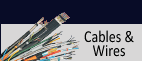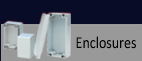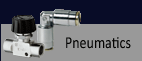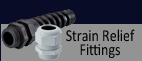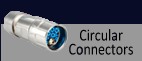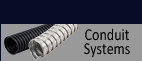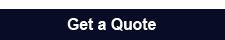*E-mail *Name *Company *Phone *State *Zip Code *Country
 Specify: P/N & Qty.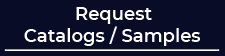303-680-5159 1-800-677-8942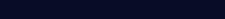## Formulas of Power Engineering

Cross-section
for direct - and single phase alternating current
(current given)
 q = 1 x I x l (mm²) κ x u for three-phase current q = 1.732 x I x cosφ x l (mm²) κ x u

for direct - and single phase alternating current
(capacity given)
 q = 2 x I x P (mm²) κ x u x U for three-phase current q = l x P (mm²) κ x u x U

Voltage drop
For low voltage cable network of normal operation, a voltage drop of 3-5% is adviseable.
Exeption: higher values (7%) can be permitted in case of network extension or in short circuit.

for direct current (current given)
 u = 2 x I x l (V) κ x q

single phase alternating current (current given)
 u = 2 x I x cosφ x l (V) κ x q

for three-phase current (current given)
 u = 1.732 x I x cosφ x l (V) κ x q

for direct current (capacity given)
 u = 2 x l x P (V) κ x q x U

single phase alternating current (capacity given)
 u = 2 x l x P (V) κ x q x U

for three-phase current (capacity given)
 u = l x P (V) κ x q x U

 u = Voltage drop (V) U = Operating voltage (V) P = Power (W) RW = Effective resistance (Ω/km) L = Inductance (mH/km) ωL = Inductive resistance (Ω/km) ω = 2 π f at 50 Hz = 314 q = Cross-section (mm²) I = Working current (A) l = Length of line (m) κ = Electrical conductivity of conductors (m/Ω x mm²) κ Copper: 58 κ Aluminum: 33
Nominal voltage
The nominal voltage is to be expressed with two values of alternating current U0/U (in V).
 U0 = voltage between conducter and ground or metallic covering (shield, armoring, concentric conductor) U = voltage between two outer conductors U0 = U/√3 for three-phase current systems U0 = U/2 for single phase and direct current systems U0/U0 = 1 outer conductor is grounded for single phase and direct current systems
Nominal current
I in A

Active current
IW = I x cosφ

Reactive current
I0 = I x sin φ

Active power
S = U x I                             for single phase current
S = 1.732 x U x I                 for three-phase current

Apparent power
P = U x I x cosφ                   for single phase current
P = 1.732 x U x I x cos φ      for three-phase current
P = U x I                             for direct current

Reactive power
Q = U x I x sin φ                 for single phase current
Q = 1.732 x U x I x sin φ     for three-phase current
(Voltampere reactive)           Q = P x tan φ

Phase angle
φ is a phase angle between voltage and current
 cos φ = 1 0.9 0.8 0.7 0.6 0.5 sin φ = 0 0.44 0.6 0.71 0.8 0.87
Insulation resistance
 RInsu = SInsu x In Da x 10-8 (MΩ x km) l d

Specific insulation resistance
 RS = R x 2π x l x 108 In Da di
 Da = Outer diameter over insulation d = Conductor diameter di = Inner diameter of insulation l = Length of the line SInsu = Spec. resistance of insulation materials (Ω x cm)
Mutual capacity
for single conductor, three-cond. and H-cable
 CB = ξr x 10³ (nF/km) 18 In Da d

Inductance
single-phase
 0.4 x ( In Da + 0.25)    mH/km r

three-phase
 0.2 x ( In Da + 0.25)    mH/km r
 Da = Distance mid to mid of both conductors r = Conductor radius ξr = Dielectric constant 0.25 = Factor for low frequency
Ground capacitance
EC = 0.6 x CB

Charging current
(only for three-phase)
ILad = U x 2 π f x CB x 10-6 (A/km per conductor at 50Hz)

Charging power

Leakage and loss factor
G = tanδ x ωC(S)
tanδ = G/ωC

 ω = 2 π f C = Capacity tanδ = Loss factor S = Siemens = 1/1Ω
Dielectric loss
DV = U² U x 2 π f x CB x tan x 10-6 (W/km)

It should be noted that for the current load of the insulated cables and wires of selected cross-section, the power ratings are to be considered.
To estimate the voltage drop of insulated cables and wires with large cross-sections of single and three-phase overhead line, the active resistance as well as indictive resistance must be considered.

Formula for single phase:
U = 2 x l x I x (RW x cosφ + ωLx sinφ) x 10-3 (V)

Formula for three-phase
U = 1.732 x l x I x (RW x cosφ + ωLx sinφ) x 10-3 (V)
f at 50Hz
 tanδ PE/VPE cables ~ 0.0005 EPR ~ 0.005 Paper single conductor, three-conductor, H-cable ~ 0.003 Oil-filled and pressure cable ~ 0.003 PVC-cable ~ 0.05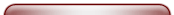Items For Quote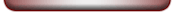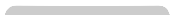Technical Information Copper & Alu Price Calculation Reference to DIN VDE Standards Harmonized Identification Code Designations Conductor Diameters and Resistance Strand Make-up acc. to DIN VDE 0295, IEC 60228 & HD 383 Nominal & Operating Voltage Current Carrying Capacity for Power Cables & Wires Installation Methods & Operating Conditions Laying Conditions for Power Cables Current Ratings for Installation Color Codes Bending Radius & Chemical Resistance Heat Resistance & Caloric Load Values Installation Instructions for UL and CSA Cables AWG Wires & AWG Stranded Conductors US and British Units - Conversions Current Ratings for UL-CSA Cables List of UL-Styles Index British Standard International Abbreviations Characteristics of Insulating and Jacket Materials Definitions: Classes of Stress in Flexible Cables and Insulated Wires Safety Requirements Glossary of Terms: Cables & Wires International Certification Marks & Testing Institute Formulas of Electronic & Power Engineering Cable-Drums - Capacity & Cable Lengths Notes on CE-Marking European Directives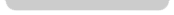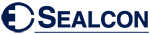is the Exclusive Importer of HUMMEL products. We offer over 6,000 different Types and Sizes of RoHS Compliant Liquid Tight Strain Relief Fittings, Cord Grips, Cable Glands, Circular Connectors, Conduit System, Industrial Enclosures and Other Related Cable Management Products which are rated the best in the industry.
7374 S. Eagle Street
Centennial, CO 80112-4221 USA
Toll Free: 800-677-8942 / 303-680-5159 FAX: 303-680-5344Top of Page